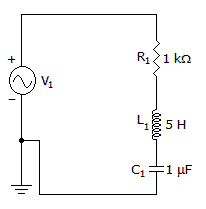# Electronics - RLC Circuits and Resonance - Discussion

### Discussion :: RLC Circuits and Resonance - General Questions (Q.No.2)

2.

What is the bandwidth of the circuit?[A]. 31.8 Hz [B]. 32.3 Hz [C]. 142 Hz [D]. 7.2 kHz

Answer: Option A

Explanation:

No answer description available for this question.

 Lalit Garg said: (Nov 1, 2010) As Q=B.W/fr so fr=1/(2pie*underroot of LC) And Q=UNDERROOT OF L/(R*UNDERROOT OF C) B.W=?

 Vimal said: (Apr 4, 2011) How?

 Maddy said: (Apr 21, 2011) @Lalit from ur formulas I got 142Hz ans. Is that ri8?

 Aysha said: (Apr 27, 2011) fr=BW*Q

 Hussain said: (Jun 1, 2011) BW = fr/Q; ---- @Aysha: i agree with u Q ---- quality factor, higher the quality factor lower the Bandwidth. Q = (1/R)*(sqrt(L/c)); fr = 1/(2*pi*sqrt(LC)); ---- resonant frequency. so BW = R/(2*pi*L); so on substituting the values BW = 31.8 Hz

 Oneidensis said: (Jun 23, 2011) My answer is 200... iIve been solving this so many times.. here's the formulas I used, f=1/(square roof of (LC)) then, Q= (1/fRC) ; Q= (fL/R) then, B=f/Q... B=200

 Sap said: (Aug 12, 2011) Hussain you are right.

 Seenivasagan said: (Sep 1, 2011) Bandwidth(BW)=fr/Q Q=(2*pi*fr*L)/R fr=1/(2*pi*root LC) by applying this , we can get fr=71.21 Q=2.236 BW=fr/Q =71.21/2.236 =31.84 approximately 31.8

 Sandeep said: (Sep 3, 2011) Q=Fr/BW; BW=Fr/Q; Fr=1/2*PI*ROOT(L*C); Q=(1/R)(ROOT(L/C));

 M.V.Krishna said: (Sep 6, 2011) BW=fr/Q; fr=1/(2*pi*underroot(L*C)); Q=(1/R)*underroot(L/C); BW=R/(2*pi*L); =>1Kohm/(2*pi*5H); =32.3Hz

 Amrita said: (Sep 17, 2011) BANDWIDTH= Resonant freq/ Quality Factor Resonant Frequency= 1/(2*pi*sqrt of(L*C)) Quality Factor= 1/R*(sqrt(L/C)) Using above fomula we can determine, Bandwidth=31.83 .

 Fadi said: (Mar 27, 2012) B.W=FR/Q Q=(1/R)SQRT(L/C) FR=1/2*PI*SQRT(LC) B.W=[1/2*PI*SQRT(LC)]/(1/R)SQRT(L/C) B.W=R*SQRT C / 2*PI*L*SQRT C B.W=R/2*PI*L

 Sreeyush Sudhakaran said: (Aug 19, 2012) B.W=fr/Q Q=(2*Pi*fr*L)/R B.W = fr/((2*Pi*fr*L)/R) = R/2*Pi*L = 1000/2*3.14*5 = 31.8Hz

 Alka said: (Jan 15, 2013) BW=fr/Q Q=(2*pi*fr*L)/R Bw=R/(2*pi*L) =31.83

 Manjunath said: (May 11, 2013) Bandwidth = R/2*pi*L . BW= 1/2*3.14*5 = 31.8 Hz.

 Govind Singh Chouhan said: (Nov 25, 2013) Data Given: R = 1k. C = 1uF. L = 5H. So, resonance freq. is = 1/2*pi*sqrt(LC). (fo is a resonance freq.) fo = 1/2*3.14*sqrt(5*1*10^-6)). fo = 1/2*3.14*sqrt(0.000005). fo = 71.42Hz. we know about the Quality factor "Q", Q = WoL/R. Q = 2*pi*fo*5/1k. Q = 2.24. The relationship between Q,fo and BW is: Q = fo/BW. So BW = fo/Q. BW = 71.42/2.24. BW = 31.88Hz.

 Shah said: (Apr 5, 2014) B' = R/L in radians. B' = 1000/5. B' = 200. B = B'/2*pi. B = 200/2*pi. B = 31.83.

 Daks said: (Oct 16, 2014) Easy way. BW = Fr/Q BW = 1/2*pi*L/R BW = R/2*pi*L BW = 31.83

 Ruben said: (Mar 27, 2015) So, when you decrease the resistance in the circuit Q will increase and bandwidth and will decrease, but phase angle theta will increase. The circuit efficiency will decrease number.

 Dhana said: (Jun 4, 2015) XL=wL. XL=(2*pi*f)(5H). XL=2*22/7*5. XL=31.42 app.

 Krupal Gowda Jedrali said: (Jul 12, 2015) = r/2.pai.L formula. = 1000/2*pai*5 = 31.8105 hz.

 Gaurav Kumar Yadav said: (Aug 19, 2015) As Bandwidth = Resonant frequency/Quality Factor. And Resonant Frequency = 1/(2*pi*Sqareroot LC). And Quality factor = 1/R*squareroot(L/C). Now BW = 1/ (2*pi*Suareroot LC)/1/R*squareroot (L/C) = R/(2*pi*L). BW = 1000/(2*3.14*5) = 31.8 Hz.

 Laurent P .Eranga said: (Nov 23, 2015) First find fr = 1/6.28 times square root of LC. You will got 71.2 hz. Then find xl = 6.28 times fl you will got 2.23 k if xl = xc. Then q = xl/r you will got 2.23 k/1 k = 2.235. If the formula for bw = fr/q. fr = 71.2 and q = 2.235 so bw = fr/q will be equals to 31.8 hz.

 Jatin Choudhary said: (Feb 21, 2016) f = R/2*3.14*L.

 Ashish said: (May 31, 2017) Here, r/2 * π * L = 31.8.

 Vijay said: (Sep 12, 2017) Q=(omega*L)/R. BW=f/Q, => BW=(f*R)/(w*L) => BW=R/(2*pi*L) => Bw=31.8.

 Mody Shaban said: (Dec 30, 2017) HOW?

 Ayush Purwar said: (Jan 23, 2018) Bandwidth= R/(2*L)...Hz. = 1000/(2*3.14*5). = 31.84 Hz.

 Suma said: (Sep 27, 2018) Here, R/(2*π*L) = 31.8.

 Pritam said: (Apr 24, 2020) Q=XL/R. BW=fr/Q. fr=1/2*pi*√LC=71.17. XL=WL=2235.87, Q=XL/R=2.23. B=71.17/2.23=31.83.

 Jyothi said: (Oct 27, 2020) BW = R/(2*π*L), BW = (1*10^3)/(2*π*5), =31.83Hz.

#### Post your comments here:

Name *:

Email   : (optional)

» Your comments will be displayed only after manual approval.

#### Current Affairs 2021

Interview Questions and Answers